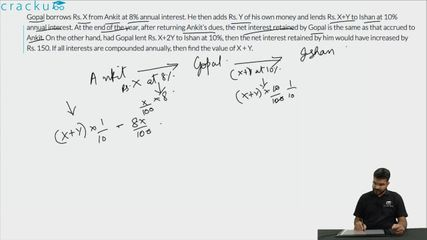Question 97

# Gopal borrows Rs. X from Ankit at 8% annual interest. He then adds Rs. Y of his own money and lends Rs. X+Y to Ishan at 10% annual interest. At the end of the year, after returning Ankit’s dues, the net interest retained by Gopal is the same as that accrued to Ankit. On the other hand, had Gopal lent Rs. X+2Y to Ishan at 10%, then the net interest retained by him would have increased by Rs. 150. If all interests are compounded annually, then find the value of X + Y.

Solution

Amount of interest paid by Ishan to Gopal if the borrowed amount is Rs. (X+Y) = $$\dfrac{10}{100}*(X+Y)$$ = 0.1(X+Y)

Gopal also borrowed Rs. X from Ankit at 8% per annum. Therefore, he has to return Ankit Rs. 0.08X as the interest amount on borrowed sum.

Hence, the interest retained by gopal = 0.1(X+Y) - 0.08X = 0.02X + 0.1Y   ... (1)

It is given that the net interest retained by Gopal is the same as that accrued to Ankit.

Therefore, 0.08X = 0.02X + 0.1Y

$$\Rightarrow$$ X = (5/3)Y   ... (2)

Amount of interest paid by Ishan to Gopal if the borrowed amount is Rs. (X+2Y) = $$\dfrac{10}{100}*(X+2Y)$$ = 0.1X+0.2Y

In this case the amount of interest retained by Gopal = 0.1X+0.2Y - 0.08X = 0.02X + 0.2Y   ... (3)

It is given that the interest retained by Gopal increased by Rs. 150 in the second case.

$$\Rightarrow$$ (0.02X + 0.2Y) - (0.02X + 0.1Y) = 150

$$\Rightarrow$$ Y = Rs. 1500

By substituting value of Y in equation (2), we can say that X = Rs. 2500

Therefore, (X+Y) = Rs. 4000.

### View Video Solution